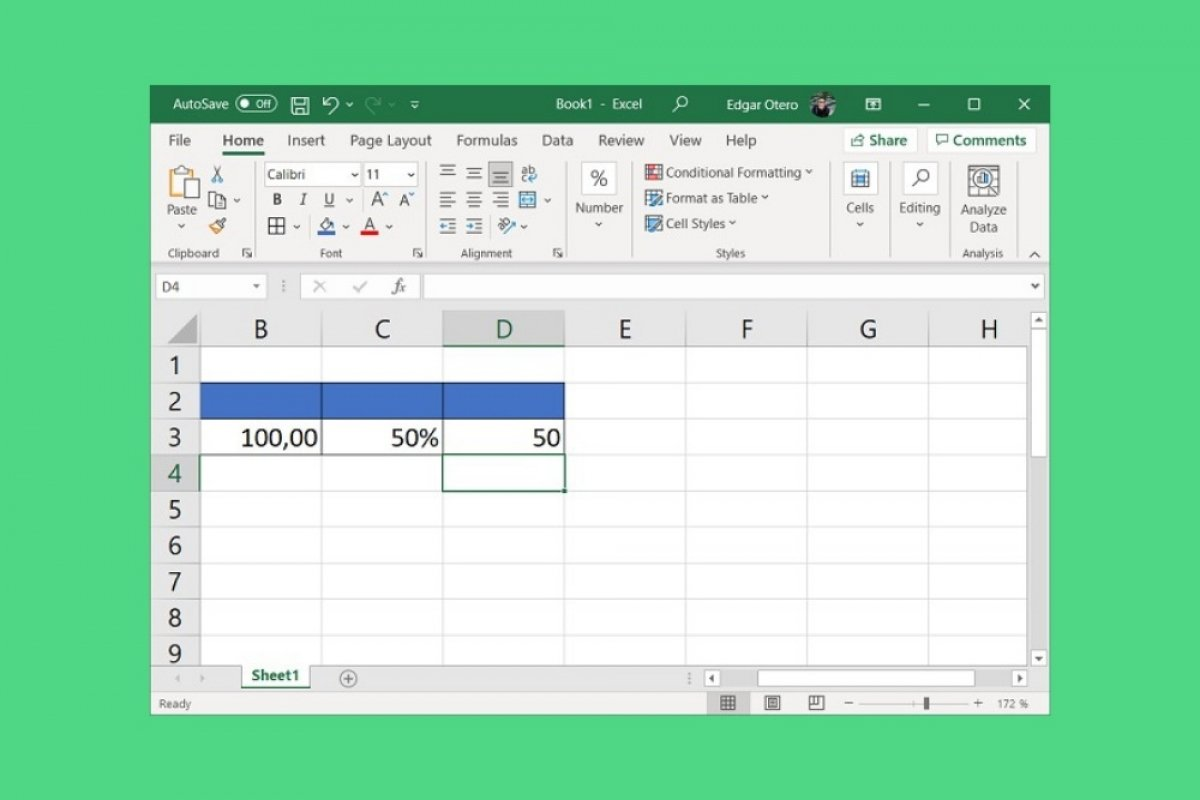One of the most basic functions of Excel is mathematical operations. Besides adding, multiplying, subtracting, or dividing, it is possible to calculate the percentage based on an initial value. There are several ways to do it, and below, we will show you all of them.

First of all, to avoid errors, you must make sure that each cell is properly formatted. This process is usually done automatically, as Excel detects what type of content is included in the cells. However, in case you need it, we tell you how to change it manually. After selecting the cell where the first piece of data is located, which is the number to extract the percentage from, click on Home, click on Number, and click on Number again.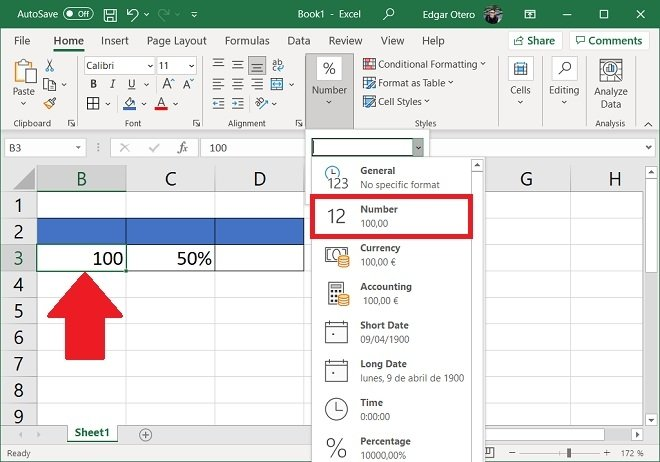Indicate the content of the cell

Repeat the process with the cell where you indicate the percentage to calculate, but in this case, select Percentage.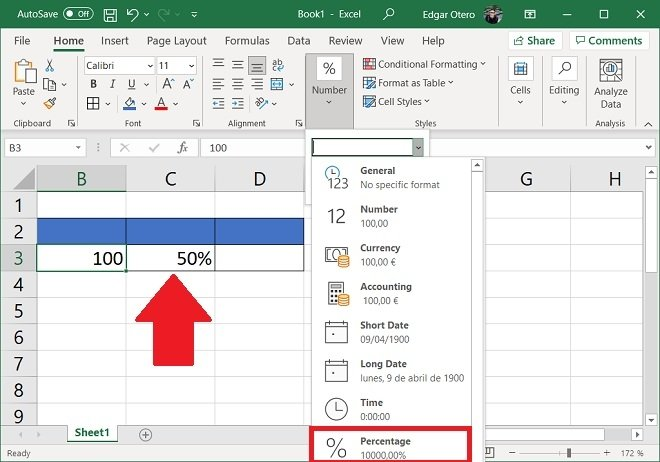Indicate that a cell is a percentage

Having specified what type of data is in each cell, it is time to make calculations.

Calculate a percentage with two cells

The first method consists of using two cells to calculate the percentage. Double-click on a blank cell, type = and select the cell of the initial value. Then, add an asterisk (*) and click on the percentage cell.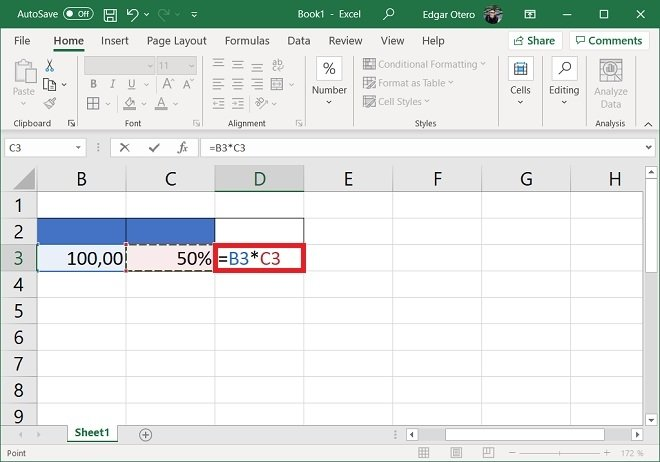Multiply two cells

Pressing Enter will immediately calculate the percentage.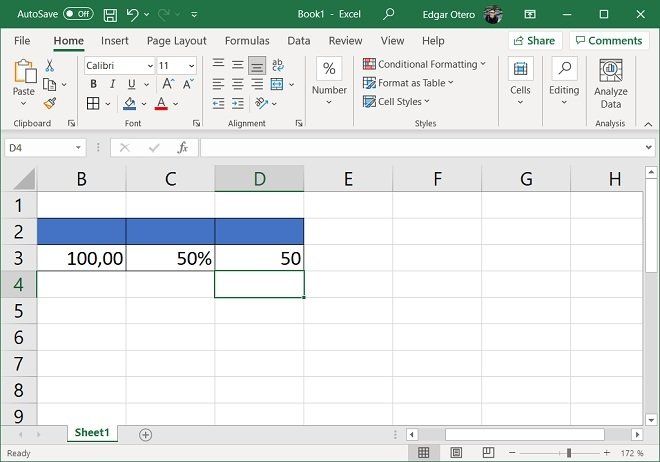Result of the multiplication

But what can you do if you do not want to use two cells to perform this calculation?

Calculate the percentage with only one initial cell

If the initial value is in a cell, but you do not want to use another cell to indicate the percentage, use the following formula:

=[initial_cell]*0.50

When you press Enter, the calculation will appear on the screen.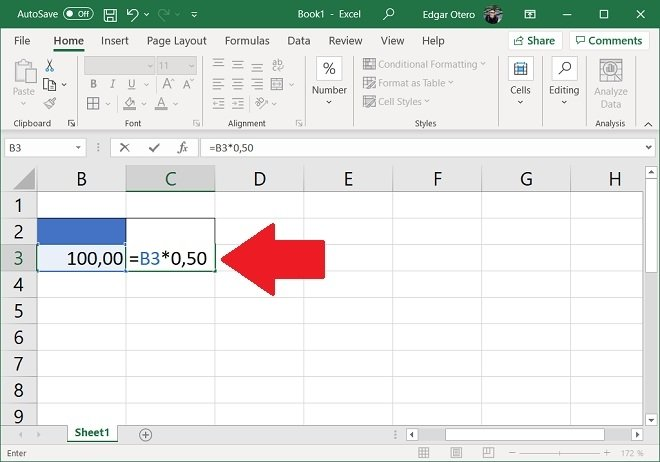Calculate without a percentage cell

This formula is also valid:

=[initial_cell]*50/100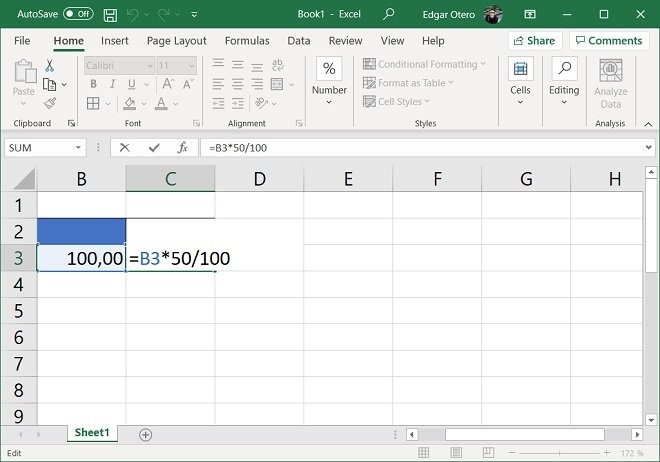Alternative formula

Finally, it is possible to use:

=[initial_cell]*50%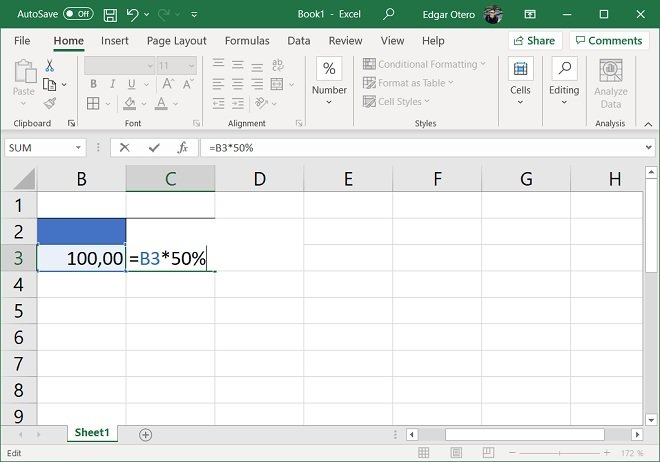Another alternative formula

All three formats create the same result.

Calculate a percentage without data cells

The last possibility is to calculate the percentage without having any cells to provide data. In this case, you should simply use any of the formulas we propose:

• =100*0,50
• =100*50/100
• =100*50%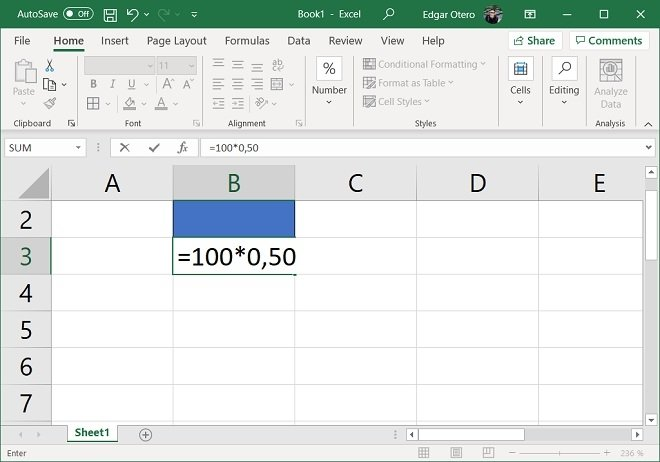Calculate a percentage without a number or percentage cell

Obviously, to obtain your own results, you need to replace the values indicated in our example with your own.